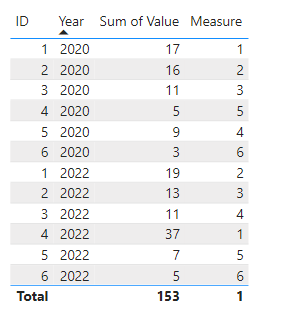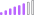cancel
Showing results for
Did you mean:Regular Visitor

## Rankx for every year

Hello Krystyna here

I am new in PowerBi world and I need some help with a RANKX function.

My data has only three columns:

ProductId, Year, Value

I need to rank products for every year. I created such measure

``ProductRank = RANKX(ALL('Table'[Year]), CALCULATE(SUM('Table'[Value]), ALLEXCEPT('Table','Table'[Year])))``

But the result is 1 for every ProductId.

What am I doing wrong?

Thanks

K

1 ACCEPTED SOLUTIONCommunity Support

Hi @Krystyna ,

Based on your description, I have created a simple sample:``````Measure =
RANKX(ALLEXCEPT('Table','Table'[Year]),CALCULATE(SUM('Table'[Value]),FILTER(ALLEXCEPT('Table','Table'[Year]),[ID]=EARLIER('Table'[ID]))),SUM('Table'[Value]),,Dense)``````

Output:Best Regards,

Jianbo Li

If this post helps, then please consider Accept it as the solution to help the other members find it more quickly.

7 REPLIES 7Community Support

Hi @Krystyna ,

Based on your description, I have created a simple sample:``````Measure =
RANKX(ALLEXCEPT('Table','Table'[Year]),CALCULATE(SUM('Table'[Value]),FILTER(ALLEXCEPT('Table','Table'[Year]),[ID]=EARLIER('Table'[ID]))),SUM('Table'[Value]),,Dense)``````

Output:Best Regards,

Jianbo Li

If this post helps, then please consider Accept it as the solution to help the other members find it more quickly.Regular Visitor

Hi Jianbo LI and thx for your help.

It works but only with Year column from this table. I use other table as a time dimension (only one column with year integer) and when I use this dimension it does not work. Could you give me a hint how should I change this measure formula? Or maybe should I add it as a column in the table rather than measure?Post Patron

@Krystyna
Hi,
Try This One

``ProductRank = RANKX(ALL('Table'[Year]), CALCULATE(SUM('Table'[Value]),,Desc)``

Thanks ,
ThennarasuRegular Visitor

Results:Many doubled ranks when value is completely differentPost Patron

@Krystyna
Rewrite Dax  Inside the ALL Functions Change column  to  Table

Thanks,
thennarasuSuper User

@Krystyna , Try like

ProductRank = RANKX(filter(ALL('Table'[Year], Table[Product]), Table[Year] = Max(Table[Year])), CALCULATE(SUM('Table'[Value])),,desc,dense)

!! Microsoft Fabric !!
Microsoft Power BI Learning Resources, 2023 !!
Learn Power BI - Full Course with Dec-2022, with Window, Index, Offset, 100+ Topics !!
Did I answer your question? Mark my post as a solution! Appreciate your Kudos !! Proud to be a Super User! !!Regular Visitor

Still all are 1